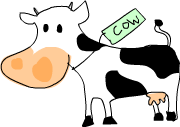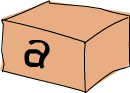# Types

## Believe the typePreviously we mentioned that Elm has a static type system. The type of every expression is known at compile time, which leads to safer code. If you write a program where you try to divide a boolean type with some number, it won’t even compile. That’s good because it’s better to catch such errors at compile time instead of having your program crash. Everything in Elm has a type, so the compiler can reason quite a lot about your program before compiling it.

Unlike Java or Pascal, Elm has type inference. If we write a number, we don’t have to tell Elm it’s a number. It can infer that on its own, so we don’t have to explicitly write out the types of our functions and expressions to get things done. We covered some of the basics of Elm with only a very superficial glance at types. However, understanding the type system is a very important part of learning Elm.

A type is a kind of label that every expression has. It tells us in which category of things that expression fits. The expression `True` is a boolean, `"hello"` is a string, etc.

Now we’ll use elm-repl to examine the types of some expressions. We’ll do that by typing expressions into elm-repl. After each valid expression, the repl tells us its type. Let’s give it a whirl.

TODO: Add a section on elm-repl, or come up with a different way to accomplish this in the online editor. Maybe type holes?

``````> 'a'
'a' : Char
> True
True : Bool
> [1, 2]
[1,2] : List number
> (True, 'a')
(True, 'a') : ( Bool, Char )
> 4 == 5
False : Bool
``````Here we see that typing an expression prints out the value of that expression followed by `:` and its type. `:` is read as “has type of”. Explicit types are always denoted with the first letter in capital case. `'a'`, as it would seem, has a type of `Char`. It’s not hard to conclude that it stands for character. `True` is of a `Bool` type. That makes sense. Examining the type of `[1, 2]` yields a `List number`. The lowercase `n` in the type of number indicates a built-in type variable. We’ll get to type variables later in this chapter. A number can be either an `Int` or a `Float`, and will concretely become one or the other depending on how it’s used. Unlike lists, each tuple length has its own type. So the expression of `(True, 'a')` has a type of `( Bool, Char )`, whereas an expression such as `('a','b','c')` would have the type of `( Char, Char, Char )`. `4 == 5` will always return `False`, so its type is `Bool`.

Functions also have types. When writing our own functions, we can choose to give them an explicit type declaration. This is generally considered to be good practice. From here on, we’ll give all the functions that we make type declarations. Remember the first function we made previously that doubles a number? Here’s how it looks like with a type declaration.

``````doubleMe : number -> number
doubleMe x =
x + x
``````

`doubleMe` has a type of `number -> number`, meaning that it maps from a number to a number. That’s because it takes one number as a parameter and returns another as a result. We didn’t have to give this function a type declaration because the compiler can infer by itself that it’s a function from a number to a number but we did anyway. But how do we write out the type of a function that takes several parameters? Here’s a simple function that takes three integers and adds them together:

``````addThree : Int -> Int -> Int -> Int
addThree x y z =
x + y + z
``````

The parameters are separated with `->` and there’s no special distinction between the parameters and the return type. The return type is the last item in the declaration and the parameters are the first three. Later on we’ll see why they’re all just separated with `->` instead of having some more explicit distinction between the return types and the parameters like `Int, Int, Int -> Int` or something.

If you want to give your function a type declaration but are unsure as to what it should be, you can always just write the function without it and then check it in elm-repl. Functions are expressions too, so it works on them without a problem. To write a multi-line expression in elm-repl, add a backslash to the end of each line you want to continue on the line below, like this.

``````> addThree x y z = \
|     x + y + z
<function> : number -> number -> number -> number
``````

Here’s an overview of some common types.

`Int` stands for integer. It’s used for whole numbers. `7` can be an `Int` but `7.2` cannot. `Int` is bounded, which means that it has a minimum and a maximum value. The maximum possible value of `Int` is `2147483647` and the `minimum` is `-2147483648`.

`Float` is a floating point with the same properties as JavaScript floats.

``````circumference : Float -> Float
circumference r =
2 * pi * r
``````
``````toPrint = circumference 4.0
25.132742
``````

`Bool` is a boolean type. It can have only two values: `True` and `False`.

`Char` represents a character. It’s denoted by single quotes.

`String` represents a string. It’s denoted by double or triple quotes and can contain many characters. Triple quoted strings are string literals that can span across multiple lines, with line breaks and whitespace being preserved in the string.

Tuples are types but they are dependent on their length as well as the types of their components, so there is theoretically an infinite number of tuple types, which is too many to cover in this tutorial. Note that the empty tuple `()` is also a type which can only have a single value: `()`. This value is read as `"unit"` and is the common way to denote an empty value with no specific meaning.

## Type variables

What do you think the type of the List `length` function is? Well, `length` takes a list of any type and returns its length, so what could it be? Let’s check!

``````> List.length
<function> : List a -> Int
``````Hmmm! What is this `a`? Is it a type? Remember that we previously stated that types are written in capital case, so it can’t exactly be a type. Because it’s not in capital case it’s actually a type variable. That means that `a` can be of any type. This is much like generics in other languages, only in Elm it’s much more powerful because it allows us to easily write very general functions if they don’t use any specific behavior of the types in them. Functions that have type variables are called polymorphic functions. The type declaration of `length` states that it takes a list of any type and returns an `Int` representing the length of that list.

Although type variables can have names longer than one character, we usually give them names of a, b, c, d …

Earlier in this chapter we mentioned the `number` type variable. This is a type variable built-in to Elm that specifically represents either an `Int` or a `Float`, but nothing else. There are other bult-in type variables representing other important things. `comparable` represents types that can be compared for equality with the `==` operator. This can be `String`, `Char`, `Int`, `Float`, `Time`, or a list or tuple containing only comparable values.

``````toPrint = 4 == 5
False
``````

`appendable` represents items that can be appended to each other with the `++` operator. These are `String`, `List`, and `Text`.

``````toPrint = "Hello" ++ "!"
"Hello!"
``````

Remember `Tuple.first`? It returns the first component of a pair. Let’s examine its type.

``````> Tuple.first
<function> : ( a1, a2 ) -> a1
``````

We see that `first` takes a tuple which contains two types and returns an element which is of the same type as the pair’s first component. That’s why we can use `first` on a pair that contains any two types. Note that just because `a1` and `a2` are different type variables, they don’t have to be different types. It just states that the first component’s type and the return value’s type are the same.